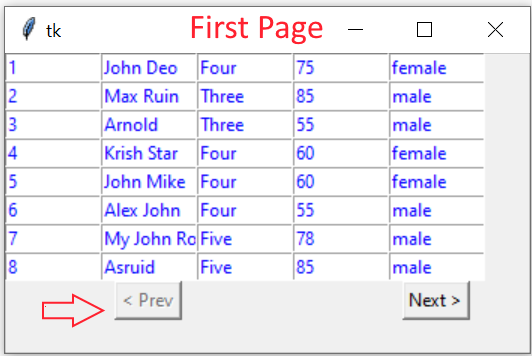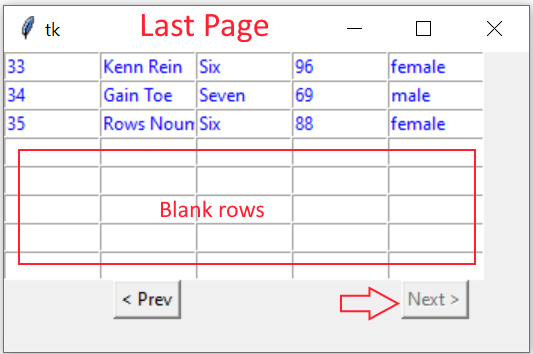# Paging script using SQLite table

Basics of Python Tkinter

## What is Paging of records ?

If we have more records then we can’t display all the records same time in one go. For example we have 35 records in our student table. We will display 10 records at a time and then give option to display next 10 records or previous 10 records to user. Breaking of records to multiple pages is known as Paging of records ( pagination ).

Download the .ipynb file in HTML format at the end of this tutorial. First Understand the basics of displaying records in Tkinter window here. We are extending this script.
• Requirements of the script is here
• Number of records per display is to be set through a variable ( script level ). Variable name is `limit`
• First records starting from 1 to the limit ( variable ) will be displayed.
• There will be two buttons at the end saying Prev ( Previous ) and Next .
• On Click of Previous button the records of previous (- limit ) records will be returned.
• On Click of Next button the records of Next ( + limit ) records will be returned.
• If there are no previous record to display then Previous button should be disabled.
• If there is no record left then Next button should be disabled.
• If there are less records left ( to be displayed) than the set limit then blank rows to be displayed.
Here is a screen shots of the output. Limit is set to 8 ,

the first page with Previous button disabled. Next button is enabled.The last page with Next button is disabled. Previous button is enabled. After displaying 35th records the rest of the rows are blank.First we will connect to SQLite database , we already created and added records to student table (You can download the my_db.db SQLite database and sample student table. There are 35 rows.
``````import sqlite3
my_conn = sqlite3.connect('my_db.db')``````
We need total number of records in the table. We also have to set the number of records per display. ( variable limit )
``````r_set=my_conn.execute("SELECT count(*) as no from STUDENT")
data_row=r_set.fetchone()
no_rec=data_row # Total number of rows in table
limit = 8; # No of records to be shown per page.``````
Basics of displaying Tkinter window is here
``````import tkinter  as tk
from tkinter import *
my_w = tk.Tk()
my_w.geometry("350x200") ``````
The function my_display() is called to display one set of rows. We pass the variable offset to this function. This is the value to start from where the records will be displayed or this is starting point of display. Initial value of offset is 0, On click of Next button it gets value 8 ( The variable limit is set to 8 ) , then it gets 16 , next 24 and so on. On click of Prev button this value decreases by 8 (the value of variable limit ).

## Creating the query.

Here we used LIMIT Query to get a set of rows. In the query we have to pass the values of variables limit and offset. While joining the values to SQL query we have to convert the variables to string ( from integer ) by using str() function.
``````q="SELECT * from student LIMIT "+ str(offset) +","+str(limit)
r_set=my_conn.execute(q);``````
We display each rows with data by using for loop and each data is displayed by using one Entry.

Our inner loop display each data horizontally one in each Entry.
``````i=0 # row value inside the loop
for student in r_set:
for j in range(len(student)):
e = Entry(my_w, width=10, fg='blue')
e.grid(row=i, column=j)
e.insert(END, student[j])
i=i+1``````
To show blank rows at the last page We will set the Entry value to blank string. This part is only executed when we have to display blank rows ( in last page only).
``````while (i<limit):#required to blank the balance rows if they are less
for j in range(len(student)):
e = Entry(my_w, width=10, fg='blue')
e.grid(row=i, column=j)
e.insert(END, "")
i=i+1``````

## Buttons with parameters

We are using two buttons here, Next button will display next set of records and Prev button will display previous set of rows.
These buttons on click will execute my_display(offset) function and pass the new offset value.
``````back = offset - limit # This value is used by Previous button
next = offset + limit # This value is used by Next button

b1 = tk.Button(my_w, text='Next >', command=lambda: my_display(next))
b1.grid(row=12,column=4)
b2 = tk.Button(my_w, text='< Prev', command=lambda: my_display(back))
b2.grid(row=12,column=1)``````

## Enable and disable buttons

We have calculated the total number of records and stored it in variable no_rec. This value we will compare with variable next and when it is less or equal to next then we will disable the NEXT button.
``````    if(no_rec <= next):
b1["state"]="disabled" # disable next button
else:
b1["state"]="active"  # enable next button``````
Similarly for Previous ( Prev ) button we will check the value of variable back. If the value is greater than or equal to 0 then we can keep the Previous button enabled.
``````    if(back >= 0):
b2["state"]="active"  # enable Prev button
else:
b2["state"]="disabled"# disable Prev button``````
Here is the full code. You can download the .ipynb file.
``````#https://www.plus2net.com/python/tkinter-sqlite-paging.php
import sqlite3
my_conn = sqlite3.connect('my_db.db')
###### end of connection ####
r_set=my_conn.execute("SELECT count(*) as no from STUDENT")
data_row=r_set.fetchone()
no_rec=data_row # Total number of rows in table
limit = 8; # No of records to be shown per page.
##### tkinter window ######
import tkinter  as tk
from tkinter import *
my_w = tk.Tk()
my_w.geometry("350x200")
def my_display(offset):

q="SELECT * from student LIMIT "+ str(offset) +","+str(limit)
r_set=my_conn.execute(q);
i=0 # row value inside the loop
for student in r_set:
for j in range(len(student)):
e = Entry(my_w, width=10, fg='blue')
e.grid(row=i, column=j)
e.insert(END, student[j])
i=i+1
while (i<limit): # required to blank the balance rows if they are less
for j in range(len(student)):
e = Entry(my_w, width=10, fg='blue')
e.grid(row=i, column=j)
e.insert(END, "")
i=i+1
# Show buttons
back = offset - limit # This value is used by Previous button
next = offset + limit # This value is used by Next button
b1 = tk.Button(my_w, text='Next >', command=lambda: my_display(next))
b1.grid(row=12,column=4)
b2 = tk.Button(my_w, text='< Prev', command=lambda: my_display(back))
b2.grid(row=12,column=1)
if(no_rec <= next):
b1["state"]="disabled" # disable next button
else:
b1["state"]="active"  # enable next button

if(back >= 0):
b2["state"]="active"  # enable Prev button
else:
b2["state"]="disabled"# disable Prev button
my_display(0)
my_w.mainloop()``````

## Using MySQL database

The connection string can be changed to use MySQL database.
Subscribe to our YouTube Channel here

## Subscribe

* indicates required
Subscribe to plus2netplus2net.com

 08-03-2022 Hello out there, this article helped me a lot - although I have a very slow brain - by digesting it line by line it helped me to get my own pagination problem solved. Took 3 weeks :-). Thank YOU for sharing your knowledge.

Post your comments , suggestion , error , requirements etc here